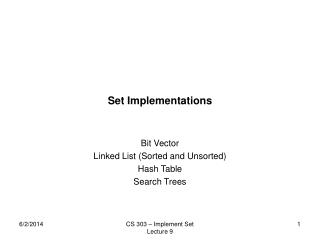DownloadDownload PresentationSet Implementations

# Set Implementations

Télécharger la présentation## Set Implementations

- - - - - - - - - - - - - - - - - - - - - - - - - - - E N D - - - - - - - - - - - - - - - - - - - - - - - - - - -
##### Presentation Transcript

1. Set Implementations Bit Vector Linked List (Sorted and Unsorted) Hash Table Search Trees CS 303 – Implement Set Lecture 9

2. Bit Vector • A = 1 0 0 1 1 0 0 1 0 1 0 0 1 1 0 1 • U = ai, 0 <= i <= 15 • A = {a0,a3,a4,a7,a9,a12,a13,a15} • 1 Bit per possible element • If |U| <= wordlength, then very efficient. If not...NOT • Easy: UNION, INTERSECTION, DIFFERENCE, MEMBER, NULL, EQUAL • Hard: MIN, MAX • Impossible: nothing – this is a “direct model” CS 303 – Implement Set Lecture 9

3. Linked List • U = ai • Unsorted: ai’s kept in any order (making insert easy? NO! why?) • Easy: NULL • Hard: Everything else! (motivation for linear order, <) • Sorted (by <): ai’s kept sorted • Hard: MEMBER • Easy: Everything else (most operations are 1 pass through data) A CS 303 – Implement Set Lecture 9

4. Dictionary ADT • MakeNull(A): A = NULL • Member(A): (x in A)? • Insert(A): A = A UNION {x} • Delete(x,A): A = A – {x} • Note: no requirement for Ordering, UNION, INTERSECTION, DIFFERENCE, ... • Applications: Phone listings, symbol tables, words (duh!) • Note that applications may SORT as a matter of implementation convenience CS 303 – Implement Set Lecture 9

5. Dictionary Implementations • Bit Vector – almost never useful • Arrays or linked list (unsorted) • MEMBER – scan entire list! • INSERT – if not MEMBER, add • DELETE – if MEMBER, remove (for Array, move everything) • All are O(n), because of MEMBER • Arrays or linked list (sorted) • speeds search (O(n) becomes O(log n) for array) • slows INSERT (O(1) becomes O(1)) • but perhaps the application doesn’t do a lot of INSERTs CS 303 – Implement Set Lecture 9

6. Binary Search Trees • An implementation technique aimed at Sets with INSERT, DELETE, MEMBER, MIN • MEMBER, MIN – Binary Search • INSERT – Search until x or L is found; add if necessary • DELETE – Find x. If a leaf, remove it. If not a leaf • find largest(smallest) y in left(right) subtree • move y to where x is • Special cases at root! [exercise!] a b c b < a < c CS 303 – Implement Set Lecture 9

7. Analysis of Binary Search Tree • Best Case: BST is balanced. All operations take O(log n) time • Worst Case: BST is linear. All operations take O(n) time • Average Case: BST is “random”. The BST is “almost balanced” and operations take • O(c log n) time. Worse than fully balanced, but only by a constant factor. • Next time – how to avoid the worst case...read about AVL, Splay, and B-trees! • But...I’ll talk about 2-3 Trees CS 303 – Implement Set Lecture 9